Courses

# Physical Equilibrium Class 11 Notes | EduRev

## Class 11 : Physical Equilibrium Class 11 Notes | EduRev

The document Physical Equilibrium Class 11 Notes | EduRev is a part of the Class 11 Course Chemistry for JEE.
All you need of Class 11 at this link: Class 11

Physical Equilibrium

Phase Transitions

There are a number of graphical means to help understand the relationships between the different phases of a particular substance. The first thing we need to do when looking at the transitions to different phases is to establish some definitions.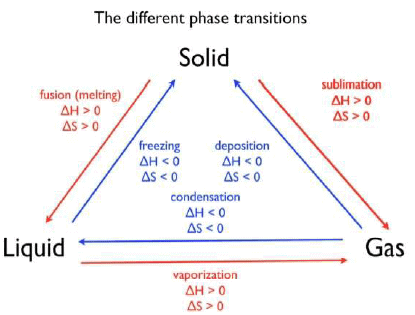There are three particular phases between which we will be examining discrete phase changes. These are solids, liquids, and gases. Each transition has a particular name.

For example, the transition from liquid to gas is called vaporization. Vaporization is endothermic, and this transition leads to an increase in entropy. The reverse transition of a gas going to a liquid is condensation. Since condensation is simply the reverse of vaporization, the changes in enthalpy and entropy will be exactly the same, but opposite in sign. So ΔHcondensation = -ΔHvaporization .

Let us consider the following physical equilibrium

H2O(l)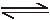H2O(g)

1Effect of temperature

Since it is an endothermic reaction therefore reaction will proceed in forward direction on increasing temp. (Le Chatelier's principle)

2Effect of pressure :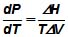for the above equilibrium ΔH > 0

ΔV ie Vg - Vl = +ve

→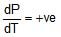→ on increasing P, T increases

But As reaction is to complete at the initial temp, therefore temperature must be lowered. This will make the reaction go to direction as suggested by Le-chatelior (endothermic reaction)

Ex. A(s) (d = 1.14 gm/cc)

B(s) (d = 1.5 gm/cc)

A(s)B(s) Heat

If mass of A and B are equal, on increasing the pressure, will for formation of B or ¯ ?

Sol. A(s)B(s)

As density of solid B is greater than solid A, VB < VA → VB - VA = - ve

since reaction is exothermic, DH = - ve

→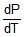=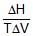,= +ve

→ On increasing pressure, the rate of formation of B will be enhanced.

LE CHATERLIER'S PRINCIPLE AND PHYSICAL EQUILIBRIUM

Consider physical equilibrium

Solid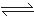Liquidvapour

i. Effect of pressure on melting

When solid melts there is a decrease in volume for some solids (ice) and increase in volume for some solid (sulphur)When ice melts there is a decrease in volume, So at constant volume there is a decrease in pressure . If pressure is increased as ice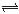water system, the equilibrium will go in forward direction. The above phenomena can also be explained from the following phase equilibrium diagram If we see phase diagram for ice water system.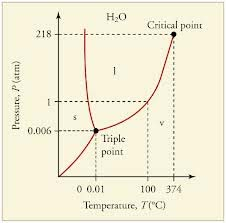So on increasing pressure, melting point is increased so equilibrium is shifted in forward direction.

ii. Vapour pressure of liquids.

Consider liquid vapour equilibrium Since evaporation of liquid is endothermic, hence rise in temperature will favour evaporation.

iii. Effect of temperature on solubility

If formation of solution is endothermic process then increase in temperature increases solubility. In formation of solution is exothermic then increase in temperature will lower the solubility.

PHASE DIAGRAM :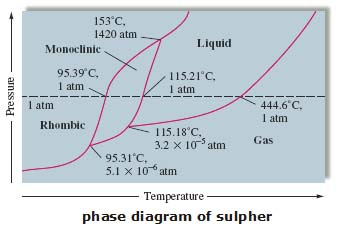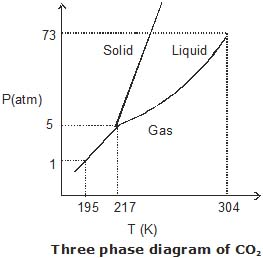Triple Point

One very special point on a phase diagram is the triple point. This is the temperature and pressure at which three phases are in equilibrium.Typically, when we are talking about a triple point it is the solid, liquid, gas triple point. For CO2this is at a pressure of 5.11 atm and -56.4 °C. Many substances have more than one solid phase, and therefore they can have more than one triple point.

Critical Point

If you follow the vaporization curve up to high temperature and pressure, you notice it simply stops. It is because this is the critical point (the on the diagram) for the substance. At temperatures and pressures greater than this point, the definition of a liquid and a gas disappear and the substance exists as a super-critical fluid (SCF). What is a super-critical fluid? It is a fluid (it takes the shape of its container) like a liquid and a gas, but it has a density that can vary between the two extremes of the liquid and the gas. This makes SCF extremely interesting. Typically, the molecules are either very close together or very far apart. In a SCF, they can essentially be anywhere in between.

What happens when you go from a gas to a SCF Essentially nothing. That is, the boundary between the liquid and the SCF and the gas and the SCF is an imaginary line defined by the critical point. This is not a phase transition. I repeat. Going from a gas to a SCF or a liquid to a SCF is not a phase transition. Going form a liquid to a gas, there is a discrete change in enthalpy, entropy, volume, density. This is a phase transition. When you "cross the line" from a gas to an SCF, there are no discrete changes.It is a continuous change. Thus, if the diagram shows this boundary, it is usually marked with dotted lines to note it is different.

Note: It is fairly easy to make CO2 a SCF (relatively low temperature and pressure). As a result, it has wide industrial use as a SCF solvent.

Offer running on EduRev: Apply code STAYHOME200 to get INR 200 off on our premium plan EduRev Infinity!

## Chemistry for JEE

223 videos|452 docs|334 tests

,

,

,

,

,

,

,

,

,

,

,

,

,

,

,

,

,

,

,

,

,

;